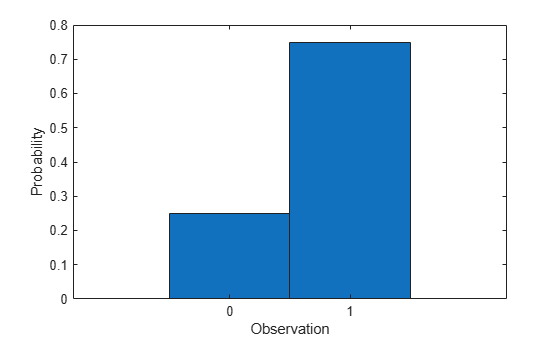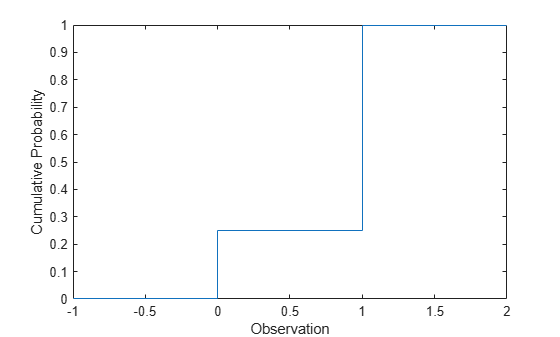## Bernoulli Distribution

### Overview

The Bernoulli distribution is a discrete probability distribution with only two possible values for the random variable. Each instance of an event with a Bernoulli distribution is called a Bernoulli trial.

### Parameters

The Bernoulli distribution uses the following parameter.

ParameterDescriptionSupport
`p`Probability of success$0\le p\le 1$

### Probability Density Function

The probability density function (pdf) of the Bernoulli distribution is

`$f\left(x|p\right)=\left\{\begin{array}{c}1-p,\text{ }x=0\\ \text{ }p,\text{ }x=1\end{array}\text{\hspace{0.17em}}.$`

For discrete distributions, the pdf is also known as the probability mass function (pmf).

For an example, see Compute Bernoulli Distribution pdf.

### Cumulative Distribution Function

The cumulative distribution function (cdf) of the Bernoulli distribution is

`$F\left(x|p\right)=\left\{\begin{array}{c}1-p,\text{ }x=0\\ \text{ }1,\text{ }x=1\end{array}\text{\hspace{0.17em}}.$`

For an example, see Compute Bernoulli Distribution cdf.

### Descriptive Statistics

The mean of the Bernoulli distribution is p.

The variance of the Bernoulli distribution is p(1 – p).

### Examples

#### Compute Bernoulli Distribution pdf

The Bernoulli distribution is a special case of the binomial distribution, where `N = 1`. Use `binopdf` to compute the pdf of the Bernoulli distribution with the probability of success `0.75`.

```p = 0.75; x = 0:1; y = binopdf(0:1,1,p);```

Plot the pdf with bars of width `1`.

```figure bar(x,y,1) xlabel('Observation') ylabel('Probability')```#### Compute Bernoulli Distribution cdf

The Bernoulli distribution is a special case of the binomial distribution, where `N = 1`. Use `binocdf` to compute the cdf of the Bernoulli distribution with the probability of success `0.75`.

```p = 0.75; y = binocdf(-1:2,1,p);```

Plot the cdf.

```figure stairs(-1:2,y) xlabel('Observation') ylabel('Cumulative Probability')```### Related Distributions

• Binomial Distribution — The binomial distribution is a two-parameter discrete distribution that models the total number of successes in repeated Bernoulli trials. The Bernoulli distribution occurs as a binomial distribution with N = 1.

• Geometric Distribution — The geometric distribution is a one-parameter discrete distribution that models the total number of failures before the first success in repeated Bernoulli trials.

 Abramowitz, Milton, and Irene A. Stegun, eds. Handbook of Mathematical Functions: With Formulas, Graphs, and Mathematical Tables. 9. Dover print.; [Nachdr. der Ausg. von 1972]. Dover Books on Mathematics. New York, NY: Dover Publ, 2013.

 Evans, Merran, Nicholas Hastings, and Brian Peacock. Statistical Distributions. 2nd ed. New York: J. Wiley, 1993.